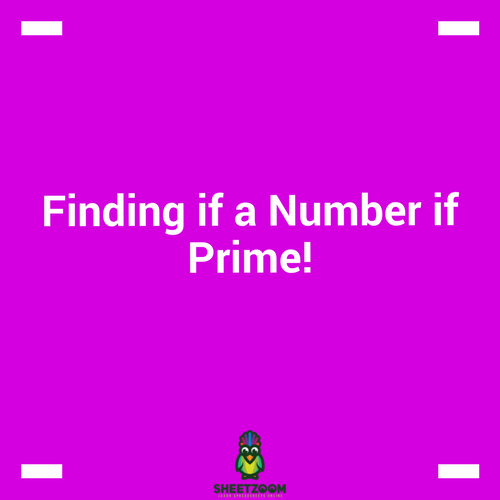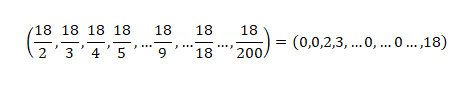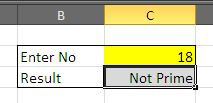# Finding if a Number if Prime!Prime Numbers are numbers that are only divisible by their selves. In this small tutorial we will guide you how to find if a number is prime or not. Just to elaborate a little the number 15 is not prime, because it can be divided by 5 and 3. However the number 17 is prime because it cannot be divided by any number except itself.

### Logic Behind the formula we are trying to make:

The prime number is only divisible by itself. If a number is perfectly divisible by any other number, the remainder must be zero. This should not be a case with Prime Number because it is not divisible by any other number hence remainder must always remain non-zero. If we count such numbers that can divide and the count comes out to be one, the number is a Prime Number!

### The formula:

If the number is present in cell B2, the following formula should give the result:

=IF(SUM((MOD(B2,ROW(A2:A200))=0)*1)=1,"Prime","Not Prime")

### Explaining Formula:

The formula can be broken down to theThe first part that is evaluated in the ROW(A2:A200). It will generate a series of numbers from 1 to 200. You can increase the number to higher values if you want, but the starting point should be A2 always. The result is the following array of Numbers:

1,2,3,4,5,…..,200

The resulting array of Numbers are feed to the MOD function. The MOD() function divides the given number with the entire array, producing yet another array of numbers like below:We equate the second equation to zero, counting zero. If the number of zeros is other then one, it implies that the number is perfectly divisible by more then one number; this means that the number is not prime number.

For our example, the number 18 is perfectly divisible by 2, 3, 9, and 18 implying it is divisible by at least 4 numbers perfectly.

At this point, the IF functions jumps in. if the number of count is one, it says “Prime”, other wise it will result in “Not Prime Number”. The final output will look like: